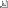PDF version of this document

Next Previous Contents

## 2. Computation

• board_map make_nth_best_move ( board_map inputboard, int color, int priority )
Description:

makes the Nth best move for color given N

Input:

the board that is dealt with, the color for which the move is carried out and the priority n where 1 is best choice

Returns:

the board after the move was carried out

• int get_mobility_of_color ( board_map inputboard, int color )
Description:

gets the number of moves available

Input:

the board that is dealt with and the color for which the number is calculated

Returns:

the number of moves available

• int evaluate_straight_lines_complete ( board_map input_board, int color )
Description:

calculate the value of the complete straight lines on the given board

Input:

the board that is dealt with and the color for which the value is calculated

Returns:

the value of the lines

• int evaluate_diagonal_lines_complete ( board_map input_board, int color )
Description:

calculate the value of the complete diagonal lines on the given board

Input:

the board that is dealt with and the color for which the value is calculated

Returns:

the value of the lines

• int evaluate_straight_lines_incomplete ( board_map input_board, int color )
Description:

calculate the value of the incomplete straight lines on the given board

Input:

the board that is dealt with and the color for which the value is calculated

Returns:

the value of the lines

• int evaluate_diagonal_lines_incomplete ( board_map input_board, int color )
Description:

calculate the value of the incomplete diagonal lines on the given board

Input:

the board that is dealt with and the color for which the value is calculated

Returns:

the value of the lines

• int evaluate_lines ( board_map input_board, int color )
Description:

calculates the value of the line occupancy for color gap in a given board

Input:

the board that is dealt with and the color for which the value is calculated

Returns:

the value required

• int calculate_mobility_difference_of_board ( board_map inputboard, int color )
Description:

calculates the mobility value gap in a given board

Input:

the board that is dealt with and the color for which the advantage is calculated

Returns:

the mobility gap in board (positive if color's mobility is higher)

• int calculate_score_difference_of_board ( board_map inputboard, int color )
Description:

calculates the score gap in a board

Input:

the board that is dealt with and the color for which the advantage is calculated

Returns:

the score gap in board (positive if color's score is higher)

• int find_value_of_position ( int i, int j, int color )
Description:

finds the value of position i,j standing for A-H and 1-8.

Input:

the board coordinates ranging from 1-8 and the current color for randomisation reasons

Returns:

the value of the position inquired

• int evaluate ( int color, board_map input_board )
Description:

evaluates the current position of the board from the point of view of color and assumes that color is currently up

Input:

the color for which the evaluation is carried out and the state of the board

Returns:

the evaluation value

• board_map compute_move_for_color ( board_map inputboard, int color )
Description:

computes a complex move for a given colour

Input:

the colour of player whose turn it is, input board

Returns:

the board with the new stone put

• int random_number ( int N )
Description:

returns a random integer between 0 and N

• int zero_one_random ( void )
Description:

returns 0 or 1 randomly

Description:

load an opening library of Othello Master from a file given a filename

Entry:

filename is a pointer to the characters of the file to open

Returns:

true if save operation was successful, false otherwise

• void init_opening_library ( void )
Description:

initialises the opening hashtable library

• char* find_board_state_ident (void)
Description:

assigns the distinct identifier to a board state

• void cpu_move ( int color )
Description:

makes a move on behalf of color using the CPU

Next Previous Contents